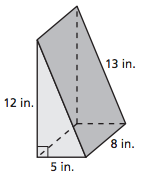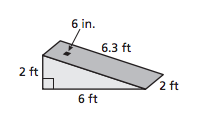# 8.2 Surface Area of PrismsMargaret Bruce
question

The sum of the areas of all of its faces. You can use a two-dimensional representation of a solid, called a net, to find the surface area of the solid.

What is surface area?
question

258 square meters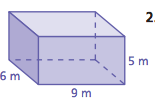Find the surface area of the rectangular prism.
question

180 square inches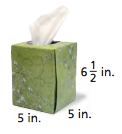Find the surface area of the rectangular prism.
question

286 square feet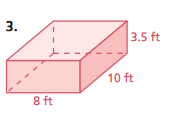Find the surface area of the rectangular prism.
question

60 square yards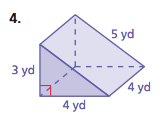Find the surface area of the triangular prism.
question

420 square meters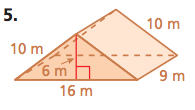question

299.32 square feet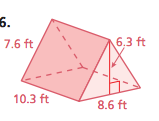Find the surface area of the triangular prism.
question

448 square inches. The surface area of the box is 448 square inches so that is the least amount of paper needed to cover the box.

A gift box is the shape of a rectangular prism measures 8 inches by 8 inches by 10 inches. What is the least amount of wrapping paper needed to wrap the gift box?
question

356 square inches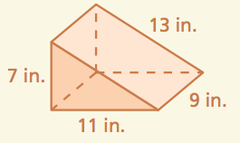Find the surface area of the triangular prism.
question

137.1 square cm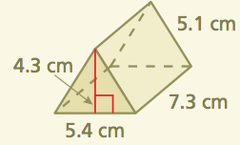Find the surface area of the triangular prism.
question

314 square meters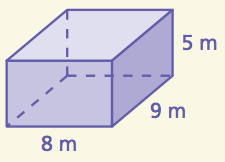Find the surface area of the rectangular prism.
question

166 square cm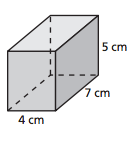question

264 square m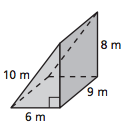question

No, you do not have enough money. You would need \$0.20 more.

You want to make a rectangular prism out of cardboard. The base is 3 feet by 4 feet. The height is 2 feet. You have \$5.00 and cardboard costs \$0.10 per square foot. Do you have enough money? If not, how much more money do you need?
question

300 square inches